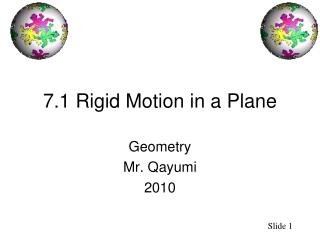DownloadDownload Presentation7.1 Rigid Motion in a Plane

# 7.1 Rigid Motion in a Plane

Download Presentation## 7.1 Rigid Motion in a Plane

- - - - - - - - - - - - - - - - - - - - - - - - - - - E N D - - - - - - - - - - - - - - - - - - - - - - - - - - -
##### Presentation Transcript

1. 7.1 Rigid Motion in a Plane Geometry Mr. Qayumi 2010

2. Standard/Objectives Standard: • Students will understand geometric concepts and applications. Performance Standard: • Describe the effect of rigid motions on figures in the coordinate plane and space that include rotations, translations, and reflections Objective: • Identify the three basic rigid transformations.

3. Assignments • Chapter 7 Definitions ( Will post online) • p. 399 – 401 #’s 1- 31, 33-39

4. TransformationsVocabulary • The new figures is called the IMAGE. • The original figures is called the PREIMAGE. • The operation that MAPS, or moves the PREIMAGE onto the image is called a TRANSFORMATION.

5. What will you learn? • Three basic transformations: • Reflections • Rotations • Translations • And combinations of the three. • For each of the three transformations on the next slide, the blue figure is the preimage and the red figure is the image. We will use this color convention throughout the rest of the book.

6. Three Transformations: Rotation about a point Reflection in a line Translation

7. Some facts • Some transformations involve labels. When you name an image, take the corresponding point of the preimage and add a prime symbol. For instance, if the preimage is A, then the image is A’, read as “A prime.”

8. Use the graph of the transformation at the right. Name and describe the transformation. Name the coordinates of the vertices of the image. Is ∆ABC congruent to its image? How can you prove it? Example 1: Naming transformations

9. Name and describe the transformation. The transformation is a reflection in the y-axis. The image was obtained by flipping ∆ABC over the y-axis/ Example 1: Naming transformations

10. Name the coordinates of the vertices of the image. The cordinates of the vertices of the image, ∆A’B’C’, are A’(4,1), B’(3,5), and C’(1,1). Example 1: Naming transformations

11. Is ∆ABC congruent to its image? Yes ∆ABC is congruent to its image ∆A’B’C’. One way to show this would be to use the DISTANCE FORMULA to find the lengths of the sides of both triangles. Then use the SSS Congruence Postulate Example 1: Naming transformations

12. ISOMETRY • An ISOMETRY is a transformation that preserves lengths. Isometries also preserve angle measures, parallel lines, and distances between points. Transformations that are isometries are called RIGID TRANSFORMATIONS.

13. Is this an Isometry? This transformation appears to be an isometry. The blue parallelogram is reflected in a line to produce a congruent red parallelogram. Ex. 2: Identifying Isometries

14. Is this an Isometry? This transformation is not an ISOMETRY because the image is not congruent to the preimage Ex. 2: Identifying Isometries

15. You can describe the transformation in the diagram by writing “∆ABC is mapped onto ∆DEF.” You can also use arrow notation as follows: ∆ABC ∆DEF The order in which the vertices are listed specifies the correspondence. Either of the descriptions implies that A D, B E, and C F. Mappings Implies mapped onto

16. In the diagram ∆PQR is mapped onto ∆XYZ. The mapping is a rotation. Given that ∆PQR ∆XYZ is an isometry, find the length of XY and the measure of Z. Ex. 3: Preserving Length and Angle Measures 35°

17. SOLUTION: The statement “∆PQR is mapped onto ∆XYZ” implies that P X, Q Y, and R Z. Because the transformation is an isometry, the two triangles are congruent. So, XY = PQ = 3 and mZ = mR = 35°. Ex. 3: Preserving Length and Angle Measures 35°